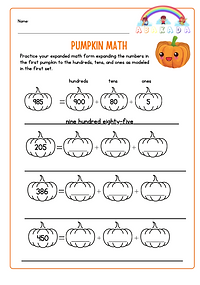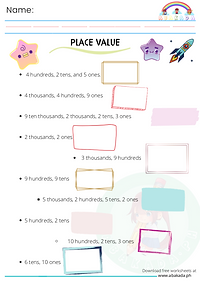top of page

# Place Value Sheets

All sheets are free for personal use.## ​## Fall Worksheet-Expanded Math

Practice your expanded math from by expanding the numbers in the first pumpkin to the hundreds, tens and ones. Write the numbers in words in the space provided.## Write the Numbers

Write the numbers and add if necessary.## Place Value: Writing in Words

Write the numerical value and place values in words.## Place Value Tens Worksheet

Write the corresponding numbers.## Place Value Tens and Ones Worksheet 2

Count the tens and ones.## Expanded Math - Candy Corns

Expand the following numbers in hundreds, tens and ones.## Place Value: Write the Numbers

Write the numbers and add if necessary.## Write the Place Values in Words

Write the numerical value and place values in words.## Place Value Hundreds Worksheet

Practice place values up to hundreds by writing the corresponding numbers.## Place Value Tens and Ones Worksheet 3

Count the tens and ones and write the numerical value.## Place Value Tens

Practice illustrating place values using this worksheet.

bottom of page